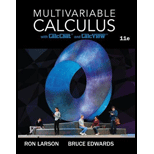# Deriving a Sum Derive Euler’s famous result that was mentioned in Section 9.3, ∑ n = 1 ∞ 1 n 2 = π 2 6 by completing each step. (a) Prove that ∫ d v 2 − u 2 + v 2 = 1 2 − u 2 arctan v 2 − u 2 + C (b) Prove that I 1 = ∫ 0 2 / 2 ∫ − u u 2 2 − u 2 + v 2 d v d u = π 2 18 by using the substitution u = 2 sin θ . (c) Prove that I 2 = ∫ 2 / 2 2 ∫ u − 2 − u + 2 2 2 − u 2 + v 2 d v d u = 4 ∫ π / 6 π / 2 arctan 1 − sin θ cos θ d θ by using the substitution u = 2 sin θ . (d) Prove the trigonometric identity 1 − sin θ cos θ = tan [ ( π / 2 ) − θ 2 ] . (e) Prove that I 2 = ∫ 2 / 2 2 ∫ u − 2 − u + 2 2 2 − u 2 + v 2 d v d u = π 2 9 . (f) Use the formula for the sum of an infinite geometric series to verify that ∑ n = 1 ∞ 1 n 2 = ∫ 0 1 ∫ 0 1 1 1 − x y d x d y . (g) Use the change of variables u = x + y 2 and v = y − x 2 to prove that ∑ n = 1 ∞ 1 n 2 = ∫ 0 1 ∫ 0 1 1 1 − x y d x d y = I 1 + I 2 = π 2 6 .### Multivariable Calculus

11th Edition
Ron Larson + 1 other
Publisher: Cengage Learning
ISBN: 9781337275378### Multivariable Calculus

11th Edition
Ron Larson + 1 other
Publisher: Cengage Learning
ISBN: 9781337275378

#### Solutions

Chapter 14, Problem 5PS
Textbook Problem

## Expert Solution

### Want to see the full answer?

Check out a sample textbook solution.

### Want to see this answer and more?

Experts are waiting 24/7 to provide step-by-step solutions in as fast as 30 minutes!*

*Response times vary by subject and question complexity. Median response time is 34 minutes and may be longer for new subjects.